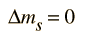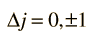# The Azimuthal Equation

Upon separation of the Schrodinger equation for the hydrogen atom, the azimuthal equation is:This is the easiest of the three separated equations to solve and gives the formBut physical constraints on the wavefunction requiresince for all integer values n, these represent the same point in space. The constants above are then required to be of the formThis is the origin of the magnetic quantum number. The expression of the separation constant in terms of this quantum number affects the form of the colatitude equation.
Index

Schrodinger equation concepts

Hydrogen concepts

 HyperPhysics***** Quantum Physics R Nave
Go Back

# The Magnetic Quantum Number

From the azimuthal equation of the hydrogen Schrodinger equation comes a quantum number with the constraintWhile the azimuthal dependence of the wavefunction only requires the quantum number to be an integer, the coupling to the colatitude equation further constrains that integer to be less than or equal to the orbital quantum number. The direct implication of this quantum number is that the z-component of angular momentum is quantized according toIt is called the magnetic quantum number because the application of an external magnetic field causes a splitting of spectral lines called the Zeeman effect. The different orientations of orbital angular momentum represented by the magnetic quantum number can be visualized in terms of a vector model.

 Quantum numbers for atomic electrons
Index

Schrodinger equation concepts

Hydrogen concepts

 HyperPhysics***** Quantum Physics R Nave
Go Back

# Selection Rules for Electronic Transitions

In spectral phenomena such as the Zeeman effect it becomes evident that transitions are not observed between all pairs of energy levels. Some transitions are "forbidden" ( i.e., highly improbable) while others are "allowed" by a set of selection rules. The number of split components observed in the Zeeman effect is consistent with the selection rules:These are the selection rules for an electric dipole transition. One can say that the oscillating electric field associated with the transitions resembles an oscillating electric dipole. When this is expressed in quantum terms, photon emission is always accompanied by a change of 1 in the orbital angular momentum quantum number. The magnetic quantum number can change by zero or one unit.

Another approach to the selection rules is to note that any electron transition which involves the emission of a photon must involve a change of 1 in the angular momentum. The photon is said to have an intrinsic angular momentum or "spin" of one, so that conservation of angular momentum in photon emission requires a change of 1 in the atom's angular momentum. The electron spin quantum number does not change in such transitions, so an additional selection rule is:The total angular momentum may change by either zero or one:An exception to this last selection rule is that you cannot have a transition from j=0 to j=0; i.e., since the vector angular momentum must change by one unit in an electronic transition, j=0 -> 0 can't happen because there is no total angular momentum to re-orient to get a change of 1.
Index

Schrodinger equation concepts

Hydrogen concepts

Atomic structure concepts

 HyperPhysics***** Quantum Physics R Nave
Go Back